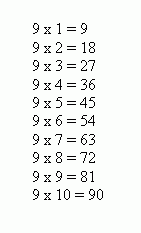Email us to get an instant 20% discount on highly effective K-12 Math & English kwizNET Programs!

#### Online Quiz (WorksheetABCD)

Questions Per Quiz = 2 4 6 8 10

### Grade 4 - Mathematics3.5 Multiplication by 9

Method 1: Using 9th table to compute the results.
An easier way of writing 9th table is shown below.
1. Writing 0 to 9 in one column.
2. Reversing the order that is 9 to 0 in the second column.
3. Read out the numbers in both the columns together.
4. This gives the multiples of 9 from 1 through 10.Example: 4 x 9

When you are multiplying by 9, on your fingers start with the thumb. Count the number you are multiplying by and hold down that finger. The number of fingers before the finger held down is the first digit of the answer and the number of finger after the finger held down is the second digit of the answer.

Example: 9 x 8
To multiply 9 x 8 on your fingers, hold up ten fingers, put down your 8th finger and the answer is 72. Example: 9 x 7
Hold up ten fingers, put down your 7th finger and the answer is 63.

Directions: Answer the following questions. Also write the multiplication facts for 9 using both the methods on a piece of paper.
 Q 1: 3 x 9=1863627 Q 2: 5 x 9=4184554 Q 3: 9 x 9=1880819 Q 4: 2 x 9=1892711 Q 5: 1 x 9=108189 Q 6: 10 x 9=901991 Q 7: 4 x 9=2736139 Q 8: 8 x 9=72746456 Question 9: This question is available to subscribers only! Question 10: This question is available to subscribers only!